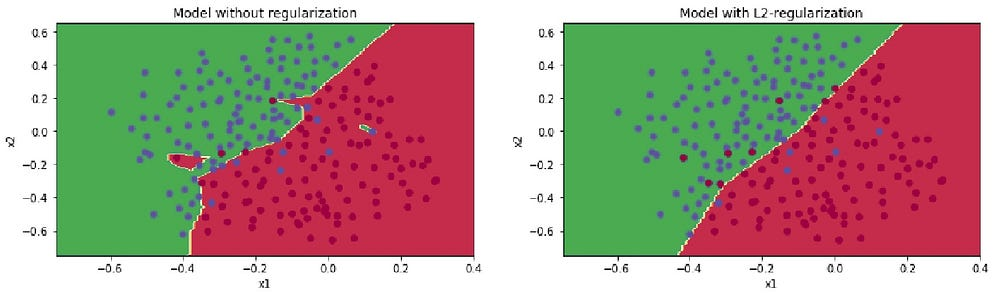# Solving overfitting in Neural Nets with Regularization

Original article can be found here (source): Deep Learning on Medium# Why Regularization reduces overfitting

When implementing regularization we added a term called Frobenius norm which penalises the weight matrices from being too large. So, now the question to think about is why does shrinking the Frobenius norm reduce overfitting?

Idea 1

An idea is that if you crank regularisation parameter λ to be really big, they’ll be really incentivized to set the weight matrices `w` to be reasonably close to zero. So one piece of intuition is maybe it set the weight to be so close to zero for a lot of hidden units that’s basically zeroing out a lot of the impact of these hidden units. And if that is the case, then the neural network becomes a much smaller and simplified neural network. In fact, it is almost like a logistic regression unit, but stacked most probably as deep. And so that would take you from the overfitting case much closer to the high bias case. But hopefully there should be an intermediate value of λ that results in an optimal solution. So, to sum up you are just zeroing or reducing out the impact of some hidden layers and essentially a simpler network.

The intuition of completely zeroing out of a bunch of hidden units isn’t quite right and does not work too good in practice. It turns out that what actually happens is we will still use all the hidden units, but each of them would just have a much smaller effect. But you do end up with a simpler network and as if you have a smaller network that is therefore less prone to overfitting.

Idea 2

Here is another intuition or idea to regularization and why it reduces overfitting. To understand this idea we take the example of `tanh` activation function. So, our `g(z) = tanh(z)` .